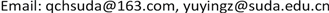1泰州学院数理学院，江苏 泰州

2苏州大学数学科学学院，江苏 苏州1. 引言

m e a s ( { x ∈ Ω : g ( x ) ≥ a } ) = m e a s ( { x ∈ Ω : f ( x ) ≥ a } ) , ∀ a ∈ R

{ − L θ s u = f ( x ) + h ( x , u ) , x ∈ Ω u = 0 , x ∈ R N \ Ω (1.1)

L θ s u ( x ) = ∫ R N u ( x + y ) + u ( x − y ) − 2 u ( x ) | y | N + 2 s θ ( y ) d y , x ∈ R N ,

( P λ , h , f ) { ( − Δ ) s u = f ( x ) + λ h ( x ) u , x ∈ Ω u = 0 , x ∈ R N \ Ω

I ( u ) = 1 2 ∫ R N ∫ R N ( u ( x ) − u ( y ) ) 2 | x − y | N + 2 s d x d y − λ 2 ∫ Ω h u 2 d x − ∫ Ω f u d x (1.2)

(Opt) I ( u h ^ , f ^ ) = inf h ∈ R ( h 0 ) , f ∈ R ( f 0 ) I ( u h , f ) ?

2. 预备知识

H s ( Ω ) = { u ∈ L 2 ( R N ) : u ≡ 0 , x ∈ R N \ Ω , ∫ R N ∫ R N ( u ( x ) − u ( y ) ) 2 | x − y | N + 2 s d x d y < ∞ } ,

〈 u , v 〉 = ∫ R N ∫ R N ( u ( x ) − u ( y ) ) ( v ( x ) − v ( y ) ) | x − y | N + 2 s d x d y , ∀ u , v ∈ H s ( Ω ) .

‖ u ‖ = ( ∫ R N ∫ R N ( u ( x ) − u ( y ) ) 2 | x − y | N + 2 s d x d y ) 1 2 .

∫ R N ∫ R N ( u ( x ) − u ( y ) ) ( v ( x ) − v ( y ) ) | x − y | N + 2 s d x d y − ∫ Ω ( λ h u v + f v ) d x = 0 , ∀ v ∈ H s ( Ω ) .

I ′ ( u ) v = ∫ R N ∫ R N ( u ( x ) − u ( y ) ) ( v ( x ) − v ( y ) ) | x − y | N + 2 s d x d y − ∫ Ω ( λ h u v + f v ) d x , ∀ v ∈ H s ( Ω ) .

,

, 若,

.

,

.

.

.

4. 重排优化问题(Opt)的可解性

,

.

,

.

.

.